# Excel formulas for conditional formatting based on another cell value

In this tutorial, we will continue exploring the fascinating world of Excel Conditional Formatting. If you do not feel very comfortable in this area, you may want to look through the previous article first to revive the basics - How to use conditional formatting in Excel.

Today are going to dwell on how to use Excel formulas to format individual cells and entire rows based on the values you specify or based on another cell's value. This is often considered advanced aerobatics of Excel conditional formatting and once mastered, it will help you push the formats in your spreadsheets far beyond their common uses.

## Excel formulas for conditional formatting based on cell value

Excel's pre-defined conditional formatting rules are mainly purposed to format cells based on their own values or the values you specify. I am talking about Data Bars, Color Scales, Icon Sets and other rules available to you on the Conditional Formatting button click.

If you want to apply conditional formatting based on another cell or format the entire row based on a single cell's value, then you will need to use Excel formulas. So, let's see how you can make a rule using a formula and after that I will provide a number of formula examples for different tasks.

### How to create a conditional formatting rule using a formula

To set up a conditional formatting rule based on a formula in Excel 2019, Excel 2016, Excel 2013 and Excel 2010, carry out these steps:

1. Select the cells you want to format. You can select one column, several columns or the entire table if you want to apply your conditional format to rows.

Tip. If you plan to add more data in the future and you want the conditional formatting rule to get applied to new entries automatically, you can either:

• Convert a range of cells to a table (Insert tab > Table). In this case, the conditional formatting will be automatically applied to all new rows.
• Select some empty rows below your data, say 100 blank rows.
2. On the Home tab, in the Styles group, click Conditional formatting > New Rule…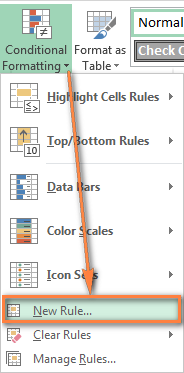3. In the New Formatting Rule window, select Use a formula to determine which cells to format.
4. Enter the formula in the corresponding box.
5. Click the Format… button to choose your custom format.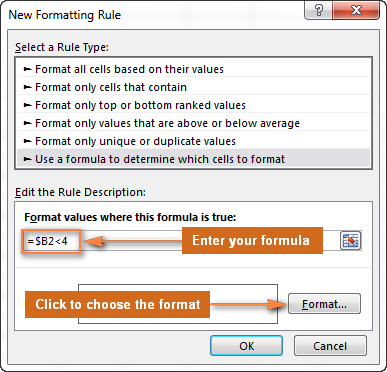6. Switch between the Font, Border and Fill tabs and play with different options such as font style, pattern color and fill effects to set up the format that works best for you. If the standard palette does not suffice, click More colors… and choose any RGB or HSL color to your liking. When done, click the OK button.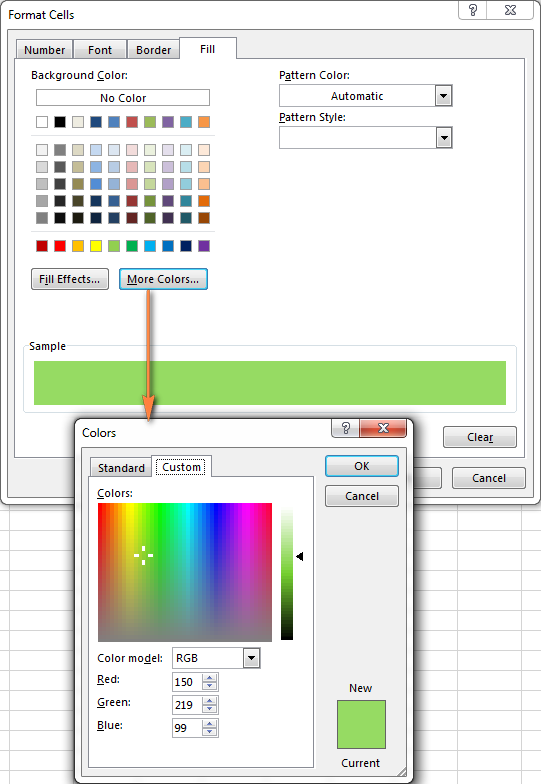7. Make sure the Preview section displays the format you want and if it does, click the OK button to save the rule. If you are not quite happy with the format preview, click the Format… button again and make the edits.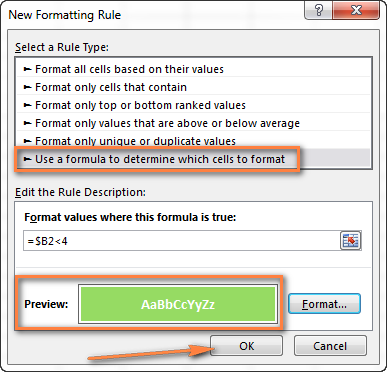Tip. Whenever you need to edit a conditional formatting formula, press F2 and then move to the needed place within the formula using the arrow keys. If you try arrowing without pressing F2, a range will be inserted into the formula rather than just moving the insertion pointer. To add a certain cell reference to the formula, press F2 a second time and then click that cell.

## Excel conditional formatting formula examples

Now that you know how to create and apply Excel conditional formatting based on another cell, let's move on and see how to use various Excel formulas in practice:

### Formulas to compare values (numbers and text)

As you know Microsoft Excel provides a handful of ready-to-use rules to format cells with values greater than, less than or equal to the value you specify (Conditional Formatting >Highlight Cells Rules). However, these rules do not work if you want to conditionally format certain columns or entire rows based on a cell's value in another column. In this case, you use analogous formulas:

 Condition Formula example Equal to `=\$B2=10` Not equal to `=\$B2<>10` Greater than `=\$B2>10` Greater than or equal to `=\$B2>=10` Less than `=\$B2<10` Less than or equal to `=\$B2<=10` Between `=AND(\$B2>5, \$B2<10)`

The screenshot below shows an example of the Greater than formula that highlights product names in column A if the number of items in stock (column C) is greater than 0. Please pay attention that the formula applies to column A only (\$A\$2:\$A\$8). But if you select the whole table (in our case, \$A\$2:\$E\$8), this will highlight entire rows based on the value in column C.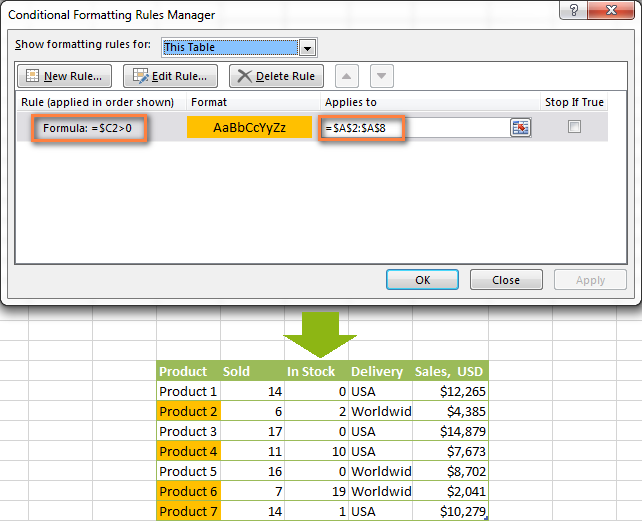In a similar fashion, you can create a conditional formatting rule to compare values of two cells. For example:

`=\$A2<\$B2` - format cells or rows if a value in column A is less than the corresponding value in column B.

`=\$A2=\$B2` - format cells or rows if values in columns A and B are the same.

`=\$A2<>\$B2` - format cells or rows if a value in column A is not the same as in column B.

As you can see in the screenshot below, these formulas work for text values as well as for numbers.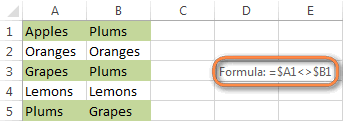### AND and OR formulas

If you want to format your Excel table based on 2 or more conditions, then use either =AND or =OR function:

 Condition Formula Description If both conditions are met `=AND(\$B2<\$C2, \$C2<\$D2)` Formats cells if the value in column B is less than in column C, and if the value in column C is less than in column D. If one of the conditions is met `=OR(\$B2<\$C2, \$C2<\$D2)` Formats cells if the value in column B is less than in column C, or if the value in column C is less than in column D.

In the screenshot below, we use the formula `=AND(\$C2>0, \$D2="Worldwide")` to change the background color of rows if the number of items in stock (Column C) is greater than 0 and if the product ships worldwide (Column D). Please pay attention that the formula works with text values as well as with numbers.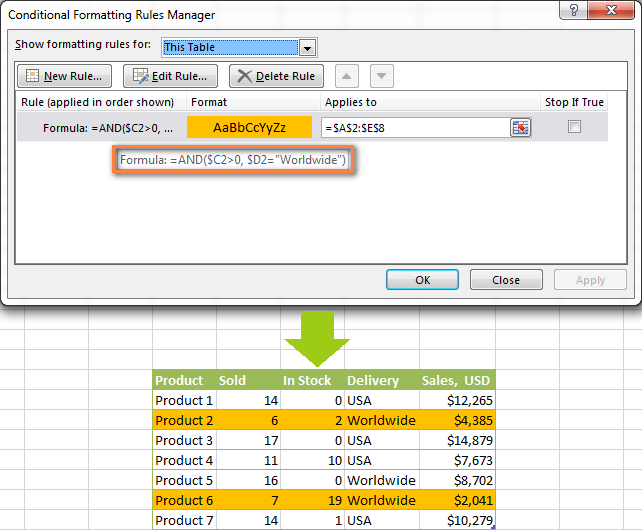Naturally, you can use two, three or more conditions in your =AND and =OR formulas.

These are the basic conditional formatting formulas you use in Excel. Now let's consider a bit more complex but far more interesting examples.

### Conditional formatting for empty and non-empty cells

I think everyone knows how to format empty and not empty cells in Excel - you simply create a new rule of the "Format only cells that contain" type and choose either Blanks or No Blanks.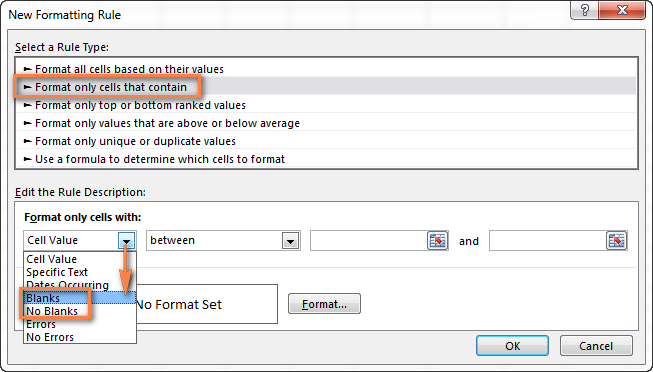But what if you want to format cells in a certain column if a corresponding cell in another column is empty or not empty? In this case, you will need to utilize Excel formulas again:

Formula for blanks: `=\$B2=""` - format selected cells / rows if a corresponding cell in Column B is blank.

Formula for non-blanks: `=\$B2<>""` - format selected cells / rows if a corresponding cell in Column B is not blank.

Note. The formulas above will work for cells that are "visually" empty or not empty. If you use some Excel function that returns an empty string, e.g. `=if(false,"OK", "")`, and you don't want such cells to be treated as blanks, use the following formulas instead `=isblank(A1)=true` or `=isblank(A1)=false` to format blank and non-blank cells, respectively.

And here is an example of how you can use the above formulas in practice. Suppose, you have a column (B) which is "Date of Sale" and another column (C) "Delivery". These 2 columns have a value only if a sale has been made and the item delivered. So, you want the entire row to turn orange when you've made a sale; and when an item is delivered, a corresponding row should turn green. To achieve this, you need to create 2 conditional formatting rules with the following formulas:

• Orange rows (a cell in column B is not empty): `=\$B2<>""`
• Green rows (cells in column B and column C are not empty): `=AND(\$B2<>"", \$C2<>"")`

One more thing for you to do is to move the second rule to the top and select the Stop if true check box next to this rule: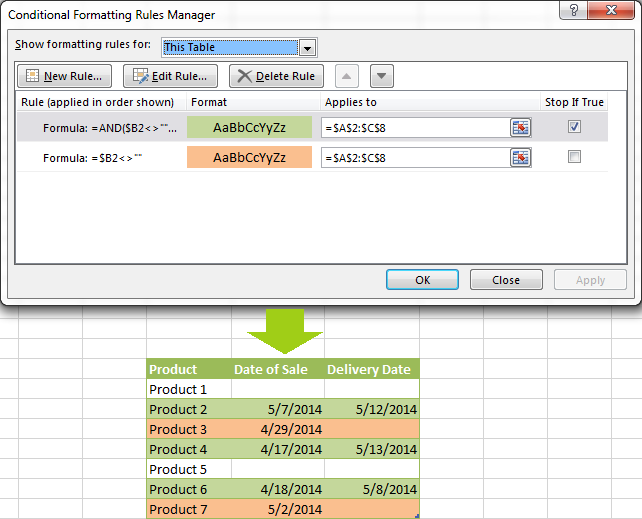In this particular case, the "Stop if true" option is actually superfluous, and the rule will work with or without it. You may want to check this box just as an extra precaution, in case you add a few other rules in the future that may conflict with any of the existing ones.

### Excel formulas to work with text values

If you want to format a certain column(s) when another cell in the same row contains a certain word, you can use a formula discussed in one of the previous examples (like =\$D2="Worldwide"). However, this will only work for exact match.

For partial match, you will need to use either SEARCH (case insensitive) or FIND (case sensitive).

For example, to format selected cells or rows if a corresponding cell in column D contains the word "Worldwide", use the below formula. This formula will find all such cells, regardless of where the specified text is located in a cell, including "Ships Worldwide", "Worldwide, except for…", etc:

`=SEARCH("Worldwide", \$D2)>0`

If you'd like to shade selected cells or rows if the cell's content starts with the search text, use this one:

`=SEARCH("Worldwide", \$D2)>1`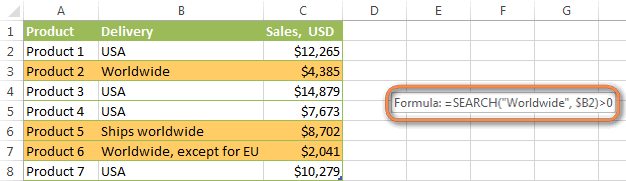### Excel formulas to highlight duplicates

If your task is to conditionally format cells with duplicate values, you can go with the pre-defined rule available under Conditional formatting > Highlight Cells Rules > Duplicate Values… The following article provides a detailed guidance on how to use this feature: How to automatically highlight duplicates in Excel.

However, in some cases the data looks better if you color selected columns or entire rows when a duplicate values occurs in another column. In this case, you will need to employ an Excel conditional formatting formula again, and this time we will be using the COUNTIF formula. As you know, this Excel function counts the number of cells within a specified range that meet a single criterion.

#### Highlight duplicates including 1st occurrences

`=COUNTIF(\$A\$2:\$A\$10,\$A2)>1` - this formula finds duplicate values in the specified range in Column A (A2:A10 in our case), including first occurrences.

If you choose to apply the rule to the entire table, the whole rows will get formatted, as you see in the screenshot below. I've decided to change a font color in this rule, just for a change : )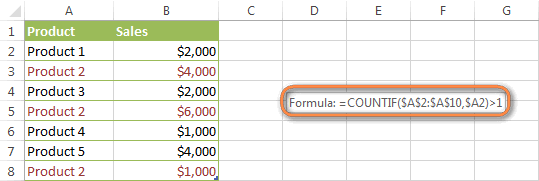#### Highlight duplicates without 1st occurrences

To ignore the first occurrence and highlight only subsequent duplicate values, use this formula: `=COUNTIF(\$A\$2:\$A2,\$A2)>1`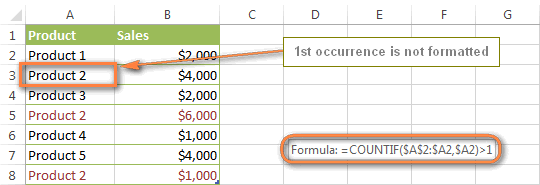#### Highlight consecutive duplicates in Excel

If you'd rather highlight only duplicates on consecutive rows, you can do this in the following way. This method works for any data types: numbers, text values and dates.

• Select the column where you want to highlight duplicates, without the column header.
• Create a conditional formatting rule(s) using these simple formulas:
Rule 1 (blue): `=\$A1=\$A2` - highlights the 2nd occurrence and all subsequent occurrences, if any.
Rule 2 (green): `=\$A2=\$A3` - highlights the 1st occurrence.

In the above formulas, A is the column you want to check for dupes, \$A1 is the column header, \$A2 is the first cell with data.

Important! For the formulas to work correctly, it is essential that Rule 1, which highlights the 2nd and all subsequent duplicate occurrences, should be the first rule in the list, especially if you are using two different colors.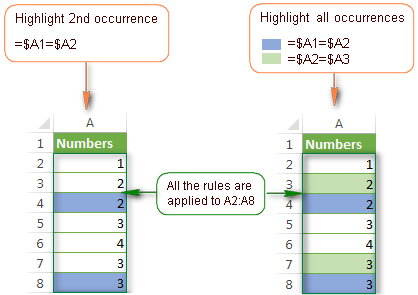#### Highlight duplicate rows

If you want apply the conditional format when duplicate values occur in two or more columns, you will need to add an extra column to your table in which you concatenate the values from the key columns using a simple formula like this one `=A2&B2`. After that you apply a rule using either variation of the COUNTIF formula for duplicates (with or without 1st occurrences). Naturally, you can hide an additional column after creating the rule.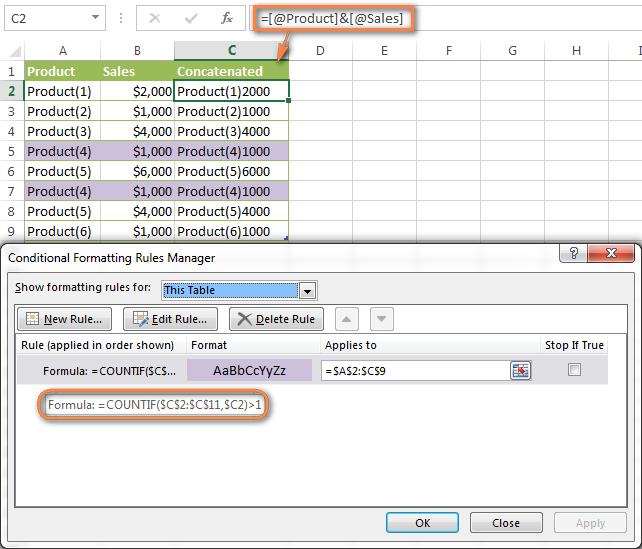Alternatively, you can use the COUNTIFS function that supports multiple criteria in a single formula. In this case, you won't need a helper column.

In this example, to highlight duplicate rows with 1st occurrences, create a rule with the following formula:
`=COUNTIFS(\$A\$2:\$A\$11, \$A2, \$B\$2:\$B\$11, \$B2)>1`

To highlight duplicate rows without 1st occurrences, use this formula:
`=COUNTIFS(\$A\$2:\$A2, \$A2, \$B\$2:\$B2, \$B2)>1`

#### Compare 2 columns for duplicates

One of the most frequent tasks in Excel is to check 2 columns for duplicate values - i.e. find and highlight values that exist in both columns. To do this, you will need to create an Excel conditional formatting rule for each column with a combination of `=ISERROR()` and `=MATCH()` functions:

For Column A: `=ISERROR(MATCH(A1,\$B\$1:\$B\$10000,0))=FALSE`

For Column B: `=ISERROR(MATCH(B1,\$A\$1:\$A\$10000,0))=FALSE`

Note. For such conditional formulas to work correctly, it's very important that you apply the rules to the entire columns, e.g. `=\$A:\$A` and `=\$B:\$B`.

You can see an example of practical usage in the following screenshot that highlights duplicates in Columns E and F.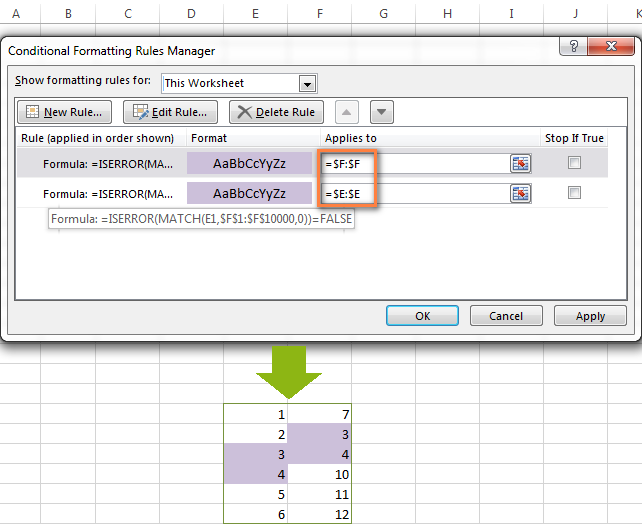As you can see, Excel conditional formatting formulas cope with dupes pretty well. However, for more complex cases, I would recommend using the Duplicate Remover add-in that is especially designed to find, highlight and remove duplicates in Excel, in one sheet or between two spreadsheets.

### Formulas to highlight values above or below average

When you work with several sets of numeric data, the AVERAGE() function may come in handy to format cells whose values are below or above the average in a column.

For example, you can use the formula `=\$E2<AVERAGE(\$E\$2:\$E\$8)` to conditionally format the rows where the sale numbers are below the average, as shown in the screenshot below. If you are looking for the opposite, i.e. to shade the products performing above the average, replace "<" with ">" in the formula: `=\$E2>AVERAGE(\$E\$2:\$E\$8)`.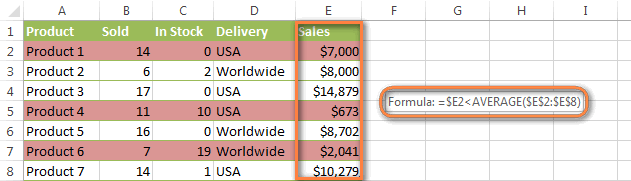### How to highlight the nearest value in Excel

If I have a set of numbers, is there a way I can use Excel conditional formatting to highlight the number in that set that is closest to zero? This is what one of our blog readers, Jessica, wanted to know. The question is very clear and straightforward, but the answer is a bit too long for the comments sections, that's why you see a solution here :)

#### Example 1. Find the nearest value, including exact match

In our example, we'll find and highlight the number that is closest to zero. If the data set contains one or more zeroes, all of them will be highlighted. If there is no 0, then the value closest to it, either positive or negative, will be highlighted.

First off, you need to enter the following formula to any empty cell in your worksheet, you will be able to hide that cell later, if needed. The formula finds the number in a given range that is closest to the number you specify and returns the absolute value of that number (absolute value is the number without its sign):

`=MIN(ABS(B2:D13-(0)))`

In the above formula, B2:D13 is your range of cells and 0 is the number for which you want to find the closest match. For example, if you are looking for a value closest to 5, the formula will change to: `=MIN(ABS(B2:D13-(5)))`

Note. This is an array formula, so you need to press Ctrl + Shift + Enter instead of a simple Enter stroke to complete it.

And now, you create a conditional formatting rule with the following formula, where B3 is the top-right cell in your range and \$C\$2 in the cell with the above array formula:

`=OR(B3=0-\$C\$2,B3=0+\$C\$2)`

Please pay attention to the use of absolute references in the address of the cell containing the array formula (\$C\$2), because this cell is constant. Also, you need to replace 0 with the number for which you want to highlight the closest match. For example, if we wanted to highlight the value nearest to 5, the formula would change to: `=OR(B3=5-\$C\$2,B3=5+\$C\$2)`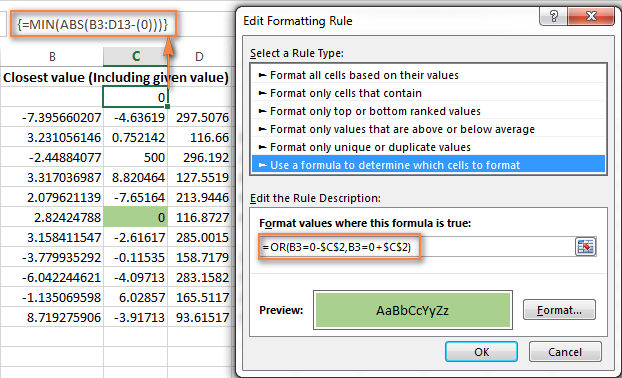#### Example 2. Highlight a value closest to the given value, but NOT exact match

In case you do not want to highlight the exact match, you need a different array formula that will find the closest value but ignore the exact match.

For example, the following array formula finds the value closest to 0 in the specified range, but ignores zeroes, if any:

`=MIN(ABS(B3:C13-(0))+(10^0*(B3:C13=0)))`

Please remember to press Ctrl + Shift + Enter after you finished typing your array formula.

The conditional formatting formula is the same as in the above example:

`=OR(B3=0-\$C\$2,B3=0+\$C\$2)`

However, since our array formula in cell C2 ignores the exact match, the conditional formatting rule ignores zeroes too and highlights the value 0.003 that is the closest match.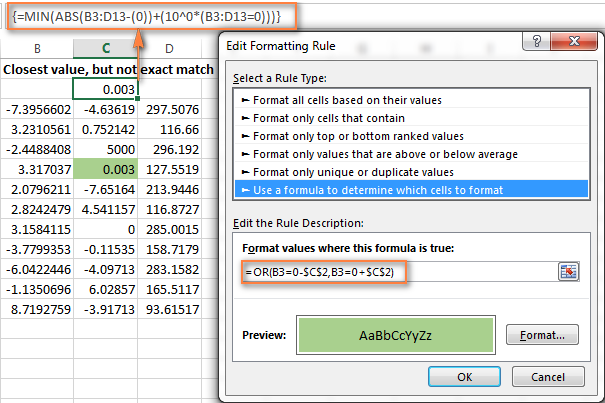If you want to find the value nearest to some other number in your Excel sheet, just replace "0" with the number you want both in the array and conditional formatting formulas.

I hope the conditional formatting formulas you have learned in this tutorial will help you make sense of whatever project you are working on. If you need more examples, please check out the following articles:

## Why isn't my Excel conditional formatting working correctly?

If your conditional formatting rule is not working as expected, though the formula is apparently correct, do not get upset! Most likely it is not because of some weird bug in Excel conditional formatting, rather due to a tiny mistake, not evident at the first sight. Please try out 6 simple troubleshooting steps below and I'm sure you will get your formula to work:

1. Use absolute & relative cell addresses correctly. It's very difficult to deduce a general rule that will work in 100 per cent of cases. But most often you would use an absolute column (with \$) and relative row (without \$) in your cell references, e.g. `=\$A1>1`.

Please keep in mind that the formulas `=A1=1`, `=\$A\$1=1` and `=A\$1=1` will produce different results. If you are not sure which one is correct in your case, you can try all : ) For more information, please see Relative and absolute cell references in Excel conditional formatting.

2. Verify the applied range. Check whether your conditional formatting rule applies to the correct range of cells. A rule of thumb is this - select all the cells / rows you want to format but do not include column headers.
3. Write the formula for the top-left cell. In conditional formatting rules, cell references are relative to the top-left most cell in the applied range. So, always write your conditional formatting formula for the 1st row with data.

For example, if your data starts in row 2, you put `=A\$2=10` to highlight cells with values equal to 10 in all the rows. A common mistake is to always use a reference to the first row (e.g. `=A\$1=10`). Please remember, you reference row 1 in the formula only if your table does not have headers and your data really starts in row 1. The most obvious indication of this case is when the rule is working, but formats values not in the rows it should.

4. Check the rule you created. Double-check the rule in the Conditional Formatting Rules Manager. Sometimes, for no reason at all, Microsoft Excel distorts the rule you have just created. So, if the rule is not working, go to Conditional Formatting > Manage Rules and check both the formula and the range it applies to. If you have copied the formula from the web or some other external source, make sure the straight quotes are used.
5. Adjust cell references when copying the rule. If you copy Excel conditional formatting using Format Painter, don't forget to adjust all cell references in the formula.
6. Split complex formulas into simple elements. If you use a complex Excel formula that includes several different functions, split it into simple elements and verify each function individually.

And finally, if you've tried all the steps but your conditional formatting rule is still not working correctly, drop me a line in comments and we will try to fathom it out together :)

In my next article we are going to look into the capabilities of Excel conditional formatting for dates. See you next week and thanks for reading!

## You may also be interested in

### 1,323 comments to "Excel formulas for conditional formatting based on another cell value"

1.Zackary says:

Hello,

I'm having trouble getting my conditional formatting to work. I have a column formatted to turn red, yellow, or green, based on how far out the date is. That part is working fine, however, I also have formatting set to grey the cell out if a separate column is marked "yes". This is working on some cells but not others and I see no reason why it shouldn't work. I have tried completely deleting all formatting and starting over. I have tried using just the grey format and it still only applies to the same cells and not the others. My formula is simple and reads =R2="yes". Do you know why this works on some cells and not others?

•Alexander Trifuntov (Ablebits Team) says:

Hello!
Unfortunately, without seeing your data it is difficult to give you any advice. Check if other conditional formatting rules apply to these cells. Also, check the values in column R. There may be extra spaces or other characters.

2.Mike C says:

I have another spreadsheet (spreadsheet 2) of about 300 people who signed up and gave their address to participate in a program to pick up material at their home for free. I want to conditionally match those addresses with addresses in spreadsheet 1 so I can locate people that signed up on a map using their latitude and longitude (this method is more accurate than other methods available).

(Address in that formula is a Named Range in spreadsheet 2, which I read enables me to compare columns from two different spreadsheets, and it worked.)

The failed matches is because people who signed up did not always write their street name the same way the county has it in its records. But they almost always get their street number correct, and the first word of the street name, so I am guessing matching about the first 12 characters will capture many more matches.

So, I want to conditionally format a match of the first 12 characters in the address column on spreadsheet 1 with the first 12 characters in the address column on spreadsheet 2.

I have tried =COUNTIFS(LEFT(Address,12),LEFT(\$O2,12)) and a number of other formulas, but all have been rejected by Excel. Help getting the correct formula would be greatly appreciated.

•Alexander Trifuntov (Ablebits Team) says:

Hello!
COUNTIFS function cannot use formula instead of a range of values. If you want to count the number of matches, use the SUMPRODUCT function.

You can also use the SEARCH function to search for matches.

3.DANIELLE BURKE says:

I need to the following to be conditionally formatted in the following manner:

1. if Column F = 4 I need the cells that have an "R" in columns G-W to be red.
2. if Column F = 3 I need the cells that have an "R" in columns G-W to be yellow.
3. If Column E = Y I need names in column C to be highlighted in Blue.

Any help would be greatly apprciated

4.Cesar joaquim Chilengue says:

Your created a Conditional Formatting Rule that will highlight the cell if any of the values in the range A3:A50 meets or exceeds the new Client Goal of 3 in cell A1, using this Rule: “=\$A\$3>=\$A\$1”. However, the formatting was changed for the entire range whether the criteria was met or not. What probably went wrong?

A. The Conditional Formatting Rule should be: =A\$3>=\$A\$1

B. The Conditional Formatting Rule should be: =\$A\$3=A1

C. The Conditional Formatting Rule should be: =\$A\$3>\$A\$1

D. The Conditional Formatting Rule should be: =A3>=\$A\$1

5.Saj says:

A1='Sheet2'!H9
when applying conditional formula in A1 as
Cell value>=\$W\$12+\$Y\$12+\$AA\$12+\$AC\$12 fill red colour
not working why?

•Saj says:

Resolved
'Sheet2'!H9 stored as text

6.Jennifer says:

I have a spread sheet where I need column (AM) to turn red if any of the information in Columns (Q), (X) or (AD) have turned red. Columns Q, X and AD are all formatted differently.

Q - Cell value is equal to NO (turns red)

X - Cell value greater than 3 (turns red)

AD - Cell value is less than -3 (turns red)

A colleague of mine had worked it out, but in his example all of the cells were formatted the same and his formula was:

=OR(A2="No",B2="No")

I'm not sure how to change it to make it work.

Any help would be greatly appreciated!

•Alexander Trifuntov (Ablebits Team) says:

Hello!
If I understand your task correctly, try the following conditional formatting formula:

Hope this is what you need.

•Jennifer says:

Thank you Alexander. I appreciate your help. The solution you provided, worked, kind of. It ended up turning cells red which should not have turned red.

But thank you for your help!

7.Christine Lawson says:

HI Have applied conditional formatting to excel spreadsheet to highlight dates of overdue , due and coming due - however even though the range is set to the entire data collection only half is working correctly - if i insert a line above the part that is not working then it will work and another line does not - it seems like only part of data will work

•Alexander Trifuntov (Ablebits Team) says:

Hi!
I don't see your data and I don't know your conditional formatting formula. So I can't give you any advice.

8.Kenneth says:

Hello,
I have a list of numbers (List A) that I want to have highlighted in another list (List B), that contains even more numbers.
I've tried to conditionally format the cells of list B by using this formula:

=COUNTIF('List A'!\$A\$2:\$A\$737,'List B'!\$A\$2:\$A\$1163)>0

on the range \$A\$2:\$A\$1163 but nothing happens.

Do you know what could be the problem?

•Kenneth says:

Thank you very much!!

9.Yuki says:

Hello.
I'm having trouble understanding conditional formatting.
If the length of a string in column Q exceeds the integer in its corresponding (same row) H column cell, I want the column Q cell in red.

The following formula works:
=LEN(Q1)>\$H1

but the following doesn't:
=LEN(Q4)>\$H4

Could you help me understand why one works while the other does not?
I feel that both should work the same, since the only difference is the row both cells are in.

•Alexander Trifuntov (Ablebits Team) says:

Hello!
Pay attention to the first paragraph of this article. Select the range of cells you want to format (ex. Q1:Q4) and then apply the rule with the first formula

10.John says:

I'm trying to use conditional formatting to highlight rows based on the contents of the first data column, i.e., if A6="Coating", then A6:J7 get formatted accordingly. In columns A:C and F:J, row 6 and 7 are merged and format properly, but D:E are not merged and only row 6 follows the rule, not 7. I have been searching for two days, but have not found the answer. I know there's a smart person out there who already knows the solution, it's just not me...

•Alexander Trifuntov (Ablebits Team) says:

Hi!
Based on cell A6, you can apply conditional formatting to only one row. You need to create a separate rule for the second row.

•John says:

I tried that to no avail. I'm not expert by any means, but I've always been able to figure it out or find the answer I've needed. I'm seriously stuck on this.

•Alexander Trifuntov (Ablebits Team) says:

Hi!
Create two conditional formatting rules separately for 6 and 7 lines. Apply both these rules to this formula: A6="Coating"
I hope this will help

11.Genie says:

Hi there, hoping you can help - i am wondering if its possible to conditionally format based on whether a cell value is present in a cell or not? I have a calculation running down column D - =((C1-\$H\$1)-B1)
but sometimes the \$H\$1 is not present and i want to hilight cells which dont use that reference. is this possible?

•Alexander Trifuntov (Ablebits Team) says:

Hi!
If you want to highlight cells that correspond to an empty cell in column H, then you can use the conditional formatting formula

=ISBLANK(H1)

If you want to highlight cells where there is a reference to \$H\$1 in the formula, you can use the conditional formatting formula

=ISNUMBER(SEARCH("\$H\$1",FORMULATEXT(D1)))

•Genie says:

Second forumla worked perfectly,

Fabulous, thank you so much for your help!

12.bea says:

Hi :)

I have a spreadsheet with the delivery status of my goods, and I'm trying to highlight the products that are delayed. So I have a column A with the week limit a good can depart and a column B with the actual date of departure of said goods.

I’m currently working with the formula ISOWEEKNUM(BF76)>BE76, but I don’t want to manually input this formula to every line of my excel sheet, but I need it to be successive, so for the next line I need it to be ISOWEEKNUM(BF77)>BE77, ISOWEEKNUM(BF78)>BE78 and so on and so forth.

Can you help ?
Thank you !!

13.Courtney says:

Hi,

I have a spreadsheet for stores with less hours with transactions than approved.

I have Column B with the hours the store should be open. With Dates from Column D to Column AE for 3 weeks worth of data.

I want to conditional format to highlight any variance to the hours in Column B that are 3 or more hours difference to the budgeted hours in Column B. So would apply to a whole table of data, but could be conditioned across so the condition stays even when I change Store #s and Dates on the sheet.

14.Jodi says:

Hi there, I have a spreadsheet where I only want to highlight the numbers greater than 0 (across 50 columns) for specific rows only (these rows have the same title "MISS"). Could you please assist with the correct formula? Thank you!

•Jodi says:

Thank you so much!

15.Belinda Young says:

I have a sheet with data in column F (formatted to number) & G (Formatted to currency) - I'm trying to highlight cells in column G that are blank or \$0.00 where there is a number in F. Anything I've tried is highlighting random cells in G.

•Alexander Trifuntov (Ablebits Team) says:

Hello!
Try using this conditional formatting formula:

=(G1=0)*ISNUMBER(F1)

16.Nidia says:

Hi
I have a main sheet that automatically updates from 3 other sheets.
I would like the cells (on main sheet) that are blank to be filled green.
For some reason it's not working, can you help?

•Alexander Trifuntov (Ablebits Team) says:

Hi!
Use conditional formatting based on cell value. New formatting rule - Format only cells that contain - Blanks.
Or use conditional formatting formula -

=ISBLANK(A1)

17.Shon says:

Hi!
I am trying to use the following:
C1 - C100 contains valued nr (quantity)
D1 - D100 contains the requested quantity
each time C# is smaller than D# i want C# to be red.
i can make it for each cell, but cant make it on all of the cells (1-100) at once instead of using the formula 100 times.
how can i make the formula work on a range of cells? (C# < D#)

•Alexander Trifuntov (Ablebits Team) says:

Hello!
When creating a conditional formatting rule, apply it directly to a range of cells. Or copy the conditional formatting, as described in this guide.

18.Manasa says:

Hello,
I have a Query,
Can i Auto Fill the Column B, based on Column A Details.

Exampls: if i mention Product 1 in Column A, It has to fill the details in column B

•Alexander Trifuntov (Ablebits Team) says:

Hi!
To automatically filling a column based on the contents of another column, try the VLOOKUP function

19.Stephan says:

Hello Team,

I have formulas that do calculations and display the result in that cell. (basic stuff)
I would like to highlight the cells depending on the results of these calculations.

When I create a rule in Conditionnal formatting, it uses the content of the cell, which is a formula. instead of using the value/result from it. Is there a way around this ?

Thanks.

•Alexander Trifuntov (Ablebits Team) says:

Hello!
Conditional formatting formulas use cell values that are obtained using calculations. You may have disabled the automatic calculation of formulas.

20.Tyler V says:

Hello,
Lets say I created an index of part locations and I named the sheet Index. It has two columns, parts and location. I created a new sheet and I want the location of the part to automatically fill when I'm looking it up. For example, I type in Part1 and in the second column Location1 is automatically filled with the correct location. I appreciate the time!

•Alexander Trifuntov (Ablebits Team) says:

Hello!
You can use the VLOOKUP function to automatically search for the desired value in a list.

21.Amanda says:

I want to know if it is possible to highlight a cell when it is one or more days before the date in another cell.
M2 = 01/02/2022
R2 = 01/04/2022

So can I highlight M2 based on it being more than one day before R2?

22.Karthik says:

I have a data. In which I want to know if A1 has 1 then D1 should be yes. If it is updated as no then the D1 cell should highlight in red. I tried many ways but was not successful. Could you please help me on this.

•Alexander Trifuntov (Ablebits Team) says:

Hello!
Please try the following conditional formatting formula:

=(A1=1)*(D1="Yes")